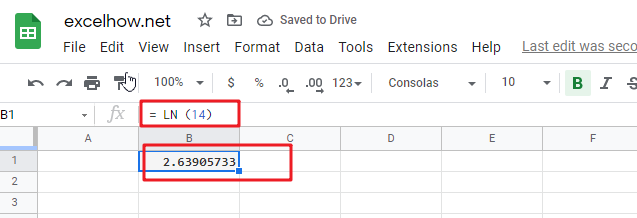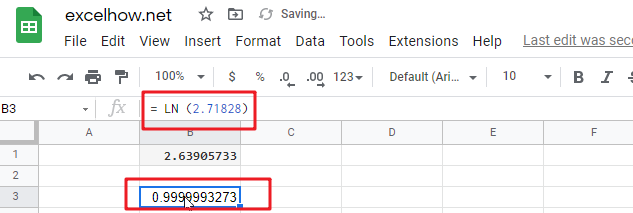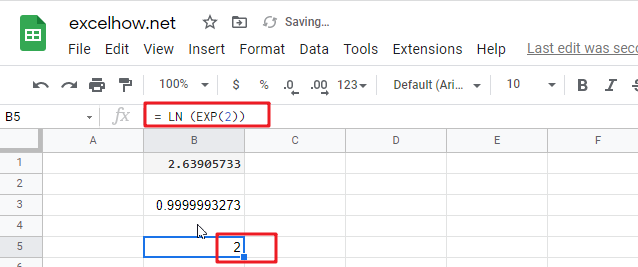This post will guide you how to use Google Sheets LN function with syntax and examples.

## Description

The Google Sheets LN function returns the natural logarithm of a given number.  And the natural logarithms are based on the constant e (the value is equal to 2.718281828…).

The purpose of this function is to get the natural logarithm of a number in google sheets. And its returned value is the natural logarithm.

The LN function is a build-in function in Google Sheets and it is categorized as a MATH function.

## Syntax

The syntax of the LN function is as below:

= LN (number)

Where the LN function arguments is:

• number – This is a required argument. The number that you want the logarithm. And the number must be a positive real number.

Note:

• If either the supplied number argument is negative or zero, the LN function will return #NUM! Error.
• If the number argument is non-numeric, the LN function will return #VALUE! Error.
• LN is the inverse of the Exp function.

## Google Sheets LN Function Examples

The below examples will show you how to use google sheets LN Function to calculate the natural logarithm of a given number.

1# to get the natural logarithm of 14, enter the following formula in Cell B1.

`= LN (14)`2# to get the natural logarithm of the value of the constant e (2.71828), enter the following formula in Cell B2.

`= LN (2.71828)`3# to get the natural logarithm of e raised to the power 2, enter the following formula in Cell B2.

= LN (EXP(2))Related Posts

This post will guide you how to use Google Sheets EXP function with syntax and examples. Description The Google Sheets EXP function returns e raised to the power of a number.  The constant e is equal to 2.71828182845904, and it ...

This post will guide you how to use Google Sheets FACT function with syntax and examples. Description The Google Sheets FACT function returns the factorial of a given number.  The factorial of a given number is equal to 1*2*3…* number. ...

This post will guide you how to use Google Sheets EVEN function with syntax and examples. Description The Google Sheets EVEN function rounds a given number up to the nearest even integer. You can use the EVEN function to return ...

This post will guide you how to use Google Sheets COUNTBLANK function with syntax and examples. Description The Google Sheets COUNTBLANK function use to count the number of empty cells in a range of cells. You can use the COUNTBLANK ...

This post will guide you how to use Google Sheets DEGREES function with syntax and examples. Description The Google Sheets DEGREES function converts radians into degrees. And it will return a numeric value. For examples, if you pass the PI() function into ...

This post will guide you how to use Google Sheets COSH function with syntax and examples. Description The Google Sheets COSH function returns the hyperbolic cosine of a given angle. The purpose of this function is used to get hyperbolic ...

This post will guide you how to use Google Sheets COS function with syntax and examples. Description The Google Sheets COS function returns the cosine of a given angle. If you want to supply an angle to COS function in ...